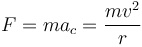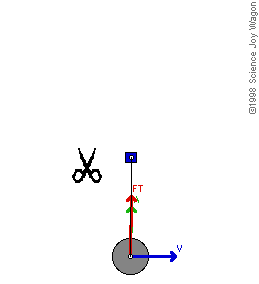Centrifugal force (Latin for "center fleeing") describes the tendency of an object following a curved path to fly outwards, away from the center of the curve. It's not really a force; it results from inertia — the tendency of an object to resist any change in its state of rest or motion. Centripetal force is a real force that counteracts the centrifugal force and prevents the object from "flying out," keeping it moving instead with a uniform speed along a circular path.

## Comparison chart

Centrifugal Force versus Centripetal Force comparison chart
Centrifugal ForceCentripetal Force
Meaning Tendency of an object following a curved path to fly away from the center of curvature. Might be described as “lack of centripetal force.” The force that keeps an object moving with a uniform speed along a circular path.
Direction Along the radius of the circle, from the center towards the object. Along the radius of the circle, from the object towards the center.
Example Mud flying off a tire; children pushed out on a roundabout. Satellite orbiting a planet
Formula Fc = mv2/r Fc = mv2/r
Defined by Chistiaan Hygens in 1659 Isaac Newton in 1684
Is it a real force? No; centrifugal force is the inertia of motion. Yes; centripetal force keeps the object from "flying out".

## Forces and Inertia

The centrifugal force is not a “real” force — the tendency to fly outwards is observed because objects that are moving in a straight line tend to continue moving in a straight line. This is called inertia, and it makes objects resistant to the force that makes them move in a curve.

The centripetal force is a "real" force. It attracts the object toward the center and prevents it from "flying out". The source of the centripetal force depends on the object in question. For satellites in orbit, the force comes from gravity. If an object is being swung around on a rope, the centripetal force is provided by tension in the rope, and for a spinning object, the force is provided by internal stress. For a car moving along an arc, the centripetal force comes from friction between the car tires and the road.

If an object is properly rotating, both the centrifugal and centripetal forces will be equal, so the object will not move towards the center of rotation or outwards from it. It will maintain a constant distance from the center.

## Direction

The centripetal force is directed inwards, from the object to the center of rotation. Technically, it is directed orthogonal to the velocity of the body, toward the fixed point of the instantaneous center of curvature of the path.

The centrifugal force is directed outwards; in the same direction as the velocity of the object. For circular motion, the velocity at any given point in time is at a tangent to the arc of movement.

## Formula

Both forces are calculated using the same formula:$F = ma_c = \frac{m v^2}{r}$

where ac is the centripetal acceleration, m is the mass of the object, moving at velocity v along a path with radius of curvature r.

## Centrifugal vs. Centripetal Force Examples

Some common examples of centrifugal force at work are mud flying off a tire and children feeling a force pushing them outwards while spinning on a roundabout.

A major example of centripetal force is the rotation of satellites around a planet.Illustration of centripetal force (red vector labeled FT, the force of tension in the rope). When the rope is cut, the centripetal force (tension in the rope) will no longer act on the object. So it will no longer be kept in that circular path by FT and will fly off on a tangent.

## Applications

Knowledge of centrifugal and centripetal forces can be applied to many everyday problems. For example, it is used when designing roads to prevent skidding and improve traction on curves and access ramps. It also allowed for the invention of the centrifuge, which separates particles suspended in fluid by spinning test tubes at high speeds.